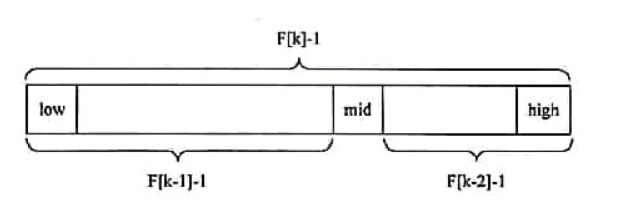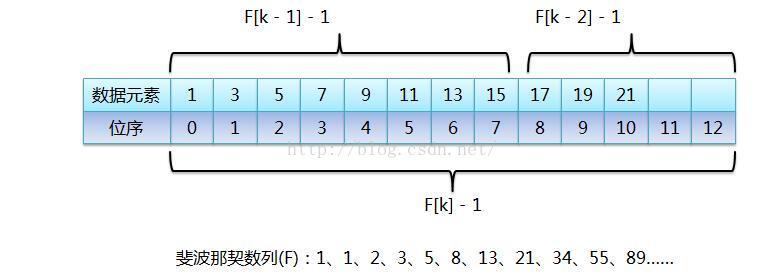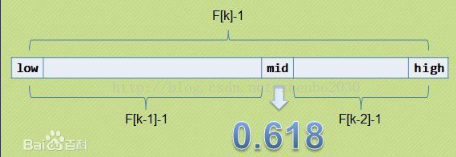• 斐波那契查找 (Fibonacci Search)
算法 - 查找 - 斐波那契查找 (Fibonacci Search)
返回分类：全部文章 >> 基础知识
返回上级：算法 - 查找与排序 (Searching and Sorting)
本文将用C++实现通用模板斐波那契查找算法，复制代码直接可使用。
在查看本文之前，需要一些程序语言的基础。
还需要熟悉 算法 - 查找 - 二分查找 (Binary Search) 。

文章目录
算法 - 查找 - 斐波那契查找 (Fibonacci Search)1 斐波那契查找简述 (Introduction)2 整型查找 (Interger Search)3 模板查找 (Template Search)4 修改整型查找 (Modify Interger Search)5 自定义类型的调用 (Custom Type)

1 斐波那契查找简述 (Introduction)
斐波那契查找，是二分查找的一个变种。其时间复杂度 O(log2n) 。
斐波那契数列，又称黄金分割数列，

F

=

{

1

,

1

,

2

,

3

,

5

,

8

,

13

,

21

,

34

,

.

.

.

}

F = \{ 1, 1, 2, 3, 5, 8, 13, 21, 34, ... \}

数学表达式：

F

0

=

1

F

1

=

1

F

2

=

F

0

+

F

1

⋮

F

i

=

F

i

−

2

+

F

i

−

1

(

i

≥

2

)

\begin{aligned} F_0 &amp;= 1 \\ F_1 &amp;= 1 \\ F_2 &amp;= F_0 + F_1 \\ &amp; \vdots \\ F_i &amp;= F_{i-2} + F_{i-1} \quad (i \geq 2) \end{aligned}

之所以它又称为黄金分割数列，是因为它的前一项与后一项的比值随着数字数量的增多逐渐逼近黄金分割比值0.618。
所以斐波那契查找改变了二分查找中原有的中值 mid 的求解方式，其 mid 不再代表中值，而是代表了黄金分割点：

m

i

d

=

l

e

f

t

+

F

b

l

o

c

k

−

1

−

1

mid = left + F_{block - 1} - 1

二分查找，以一半元素来分割数组；  斐波那契查找，以黄金分割点来分割数组。
假设表中有 n 个元素，查找过程为取区间中间元素的下标 mid ，对 mid 的关键字与给定值的关键字比较：
（1）如果与给定关键字相同，则查找成功，返回在表中的位置；  （2）如果给定关键字大，向右查找并减小2个斐波那契区间；  （3）如果给定关键字小，向左查找并减小1个斐波那契区间；  （4）重复过程，直到找到关键字（成功）或区间为空集（失败）。
通常情况下：
返回值，代表下标；  返回-1，代表没有找到关键字；
举例，假设有集合 e0=2, e1=3, e2=7, e3=14, e4=22, e5=33, e6=55, e7=75, e8=89, e9=123 。
查找元素44的过程：
已知：
元素数量size = 10；下标区间 [low, high] 为left = 0、right = 9。  则初始化的斐波那契数列为

F

i

b

=

{

1

,

1

,

2

,

3

,

5

,

8

,

13

}

Fib = \{ 1, 1, 2, 3, 5, 8, 13 \}

，即斐波那契数列最后一位要比size - 1大。  此时查找第一步为：此时刚初始化完，斐波那契数列最后一个区间为第6个区间，即区间(8, 13)；第8个数字为当前黄金分割点，则第8个数字下标为下标7，检测的数字为 e7 ；以8分割数组后，左区间为(1, 8)。

b

l

o

c

k

0

=

6

m

i

d

0

=

l

e

f

t

0

+

F

i

b

[

b

l

o

c

k

0

−

1

]

−

1

=

0

+

8

−

1

=

7

i

n

d

e

x

0

=

min

⁡

(

m

i

d

0

,

s

i

z

e

−

1

)

=

7

∵

(

e

=

44

)

&lt;

(

E

[

i

n

d

e

x

0

]

=

75

)

(小于，去左区间)

∴

l

e

f

t

1

=

l

e

f

t

0

=

0

r

i

g

h

t

1

=

m

i

d

0

−

1

=

6

b

l

o

c

k

1

=

b

l

o

c

k

0

−

1

=

5

\begin{array}{rl} block_0 &amp;= 6 \\ mid_0 &amp;= left_0 + Fib[block_0 - 1] - 1 \\ &amp;= 0 + 8 - 1 \\ &amp;= 7 \\ index_0 &amp;= \min(mid_0, size - 1) \\ &amp;= 7 \\ \because &amp; (e=44) &lt; (E[index_0]=75) \quad \text{(小于，去左区间)} \\ \therefore &amp; left_1 = left_0 = 0 \\ &amp; right_1 = mid_0 - 1 = 6 \\ &amp; block_1 = block_0 - 1 = 5 \end{array}

此时查找第二步为：同第一步，黄金分割点为第5个数字下标为4。

m

i

d

1

=

l

e

f

t

1

+

F

i

b

[

b

l

o

c

k

1

−

1

]

−

1

=

0

+

5

−

1

=

4

i

n

d

e

x

1

=

min

⁡

(

m

i

d

1

,

s

i

z

e

−

1

)

=

4

∵

(

e

=

44

)

&gt;

(

E

[

i

n

d

e

x

1

]

=

22

)

(大于，去右区间)

∴

l

e

f

t

2

=

m

i

d

1

+

1

=

5

r

i

g

h

t

2

=

r

i

g

h

t

1

=

6

b

l

o

c

k

2

=

b

l

o

c

k

1

−

2

=

3

(注意：区间是从left开始算，不是从0)

\begin{array}{rl} mid_1 &amp;= left_1 + Fib[block_1 - 1] - 1 \\ &amp;= 0 + 5 - 1 \\ &amp;= 4 \\ index_1 &amp;= \min(mid_1, size - 1) \\ &amp;= 4 \\ \because &amp; (e=44) &gt; (E[index_1]=22) \quad \text{(大于，去右区间)}\\ \therefore &amp; left_2 = mid_1 + 1 = 5 \\ &amp; right_2 = right_1 = 6 \\ &amp; block_2 = block_1 - 2 = 3 \\ &amp; \text{(注意：区间是从left开始算，不是从0)} \end{array}

上一步结束，还剩 { 33 55 } ，此时查找第三步为：黄金分割点为第2个数字55，它的下标为6。

m

i

d

2

=

l

e

f

t

2

+

F

i

b

[

b

l

o

c

k

2

−

1

]

−

1

=

5

+

2

−

1

=

6

i

n

d

e

x

2

=

min

⁡

(

m

i

d

2

,

s

i

z

e

−

1

)

=

6

∵

(

e

=

44

)

&lt;

(

E

[

i

n

d

e

x

2

]

=

55

)

(小于，去左区间)

∴

l

e

f

t

3

=

l

e

f

t

2

=

5

r

i

g

h

t

3

=

m

i

d

2

−

1

=

5

b

l

o

c

k

3

=

b

l

o

c

k

2

−

1

=

2

\begin{array}{rl} mid_2 &amp;= left_2 + Fib[block_2 - 1] - 1 \\ &amp;= 5 + 2 - 1 \\ &amp;= 6 \\ index_2 &amp;= \min(mid_2, size - 1) \\ &amp;= 6 \\ \because &amp; (e=44) &lt; (E[index_2]=55) \quad \text{(小于，去左区间)} \\ \therefore &amp; left_3 = left_2 = 5 \\ &amp; right_3 = mid_2 - 1 = 5 \\ &amp; block_3 = block_2 - 1 = 2 \end{array}

上一步结束，还剩 { 33 } ，此时查找第四步为：黄金分割点为第1个数字33，它的下标为5。

m

i

d

3

=

l

e

f

t

3

+

F

i

b

[

b

l

o

c

k

3

−

1

]

−

1

=

5

+

1

−

1

=

5

i

n

d

e

x

3

=

min

⁡

(

m

i

d

3

,

s

i

z

e

−

1

)

=

5

∵

(

e

=

44

)

&gt;

(

E

[

i

n

d

e

x

3

]

=

33

)

(大于，去右区间)

∴

l

e

f

t

4

=

m

i

d

3

+

1

=

6

r

i

g

h

t

4

=

r

i

g

h

t

3

=

5

b

l

o

c

k

4

=

b

l

o

c

k

3

−

2

=

0

∵

l

e

f

t

4

&gt;

r

i

g

h

t

4

∴

b

r

e

a

k

(退出循环，查找失败)

\begin{array}{rl} mid_3 &amp;= left_3 + Fib[block_3 - 1] - 1 \\ &amp;= 5 + 1 - 1 \\ &amp;= 5 \\ index_3 &amp;= \min(mid_3, size - 1) \\ &amp;= 5 \\ \because &amp; (e=44) &gt; (E[index_3]=33) \quad \text{(大于，去右区间)} \\ \therefore &amp; left_4 = mid_3 + 1 = 6 \\ &amp; right_4 = right_3 = 5 \\ &amp; block_4 = block_3 - 2 = 0 \\ \because &amp; left_4 &gt; right_4 \\ \therefore &amp; break \quad \text{(退出循环，查找失败)} \end{array}

之后的程序，我们以数组列表形式描述。
注意：代码全部使用std::vector<MyType>作为数组列表，如果你用指针数组MyType*，还需传入数组大小size。

2 整型查找 (Interger Search)
一般举例中，查找最基本的元素本身就是整型。
基本思路，循环整张表即可。
// Author: https://blog.csdn.net/DarkRabbit
// Fibonacci Search
// 整型有序表 - 斐波那契查找
// params:
//      list:       查找的有序表
//      element:    查找的元素
// return:
//      int:        找到的数字
template<typename T>
int FibonacciSearch(const std::vector<T>& list,
const T& element)
{
if (pEqual == nullptr || list.empty())
{
return -1;
}

int size = list.size(); // 列表长度

std::vector<int> fib(2, 1); // 斐波那契数列
int fibBlock = 1;
while (size > fib[fibBlock] - 1) // 一边构造数列，一边计算区间的位置
{
fibBlock++;
if (fibBlock == fib.size())
{
fib.push_back(fib[fibBlock - 1] + fib[fibBlock - 2]);
}
}

// 二分过程
int left = 0;
int right = size - 1;
int mid;
int index;
while (left <= right)
{
mid = left + fib[fibBlock - 1] - 1;
index = mid >= size ? size - 1 : mid;

if (element > list[index])
{
left = mid + 1;
fibBlock -= 2;
}
else if (element < list[index])
{
right = mid - 1;
fibBlock -= 1;
}
else
{
return index;
}
}

return -1;
}


3 模板查找 (Template Search)
在实际应用中，通常情况下，列表存储的都是一些数据（结构体或类），它们都包含唯一标识（即关键字Key）。
我们一般不会将它们的关键字重新建立一个列表，再去查找。
这在C++中通常用模板 (template) 来解决，其它语言多数用泛型 (Genericity) 来解决。
我们的要求仅仅是用结构中的关键字进行比较，即我们只关心关键字而不关心这个数据的类型。这样使用自定义类型也不怕了，所以可以使用一个函数指针传入比较方法，在函数中自定义比较。
我们规定此函数指针的结果：
返回值大于0，则给定关键字大，向右查找并减小2个斐波那契区间；  返回值等于0，成功找到，则返回结构；  返回值小于0，则给定关键字小，向左查找并减小1个斐波那契区间；
我们接下来改造成模板函数：
// Author: https://blog.csdn.net/DarkRabbit
// Fibonacci Search
// 模板有序表 - 斐波那契查找
// params:
//      list:       查找的有序表
//      element:    查找的元素
//      pEqual:     判断查找标准，
//                  >0 查找的值比斐波那契值大
//                  =0 找到了
//                  <0 查找的值比斐波那契值小
// return:
//      int:        找到的数字
template<typename T>
int FibonacciSearch(const std::vector<T>& list,
const T& element,
int (*pEqual)(const T&, const T&))
{
if (pEqual == nullptr || list.empty())
{
return -1;
}

int size = list.size(); // 列表长度

std::vector<int> fib(2, 1); // 斐波那契数列
int fibBlock = 1;
while (size > fib[fibBlock] - 1) // 一边构造数列，一边计算列表在数列的位置
{
fibBlock++;
if (fibBlock == fib.size())
{
fib.push_back(fib[fibBlock - 1] + fib[fibBlock - 2]);
}
}

// 二分过程
int left = 0;
int right = size - 1;
int isEqual;
int mid;
int index;
while (left <= right)
{
mid = left + fib[fibBlock - 1] - 1;
index = mid >= size ? size - 1 : mid;

isEqual = (*pEqual)(element, list[index]);
if (isEqual > 0)
{
left = mid + 1;
fibBlock -= 2;
}
else if (isEqual < 0)
{
right = mid - 1;
fibBlock -= 1;
}
else
{
return index;
}
}

return -1;
}


4 修改整型查找 (Modify Interger Search)
有了模板函数后，我们之前的整型函数可以进行修改，直接调用模板函数即可。
我们在这里直接传入 Lambda 表达式：
// Author: https://blog.csdn.net/DarkRabbit
// Fibonacci Search
// 整型有序表 - 斐波那契查找
// params:
//      list:       查找的有序表
//      element:    查找的元素
// return:
//      int:        找到的数字
int FibonacciSearch(const std::vector<int>& list,
const int& element)
{
return FibonacciSearch<int>(list, element,
[](const int& x, const int& y)->int
{
return x - y;
});
}


5 自定义类型的调用 (Custom Type)
类似的，自定义类型调用：
// Author: https://blog.csdn.net/DarkRabbit
// Fibonacci Search

#include <vector>
#include <string>
#include <iostream>
using namespace std;

struct MyElement
{
int key;
string data;
};

int MyFibonacciCompare(const MyElement& x,
const MyElement& y)
{
return x.key - y.key;
}

int main()
{
vector<MyElement> list; // 列表
MyElement tofind; // 需要查找的元素

// TODO 省略初始化列表和元素的过程

int index = FibonacciSearch<MyElement>(list, tofind,
[](const MyElement& x, const MyElement& y)->int
{
return x.key - y.key;
});

// 以上调用方法等同于
// int index = FibonacciSearch<MyElement>(list,
//                                        tofind,
//                                        MyFibonacciCompare);

if (index != -1)
{
// do something
cout << "找到了下标：" << index << endl;
}

return 0;
}


展开全文算法 C++
• 2019-07-17 斐波那契数列，又称黄金分割数列，因数学家列昂纳多·斐波那契以兔子繁殖为例而引入，故又称为”兔子数列“，指的是这样一个数列：1、1、2、3、5、8、13、21、34、… ；...斐波那契查找就是在二
2019-07-17

斐波那契数列，又称黄金分割数列，因数学家列昂纳多·斐波那契以兔子繁殖为例而引入，故又称为”兔子数列“，指的是这样一个数列：1、1、2、3、5、8、13、21、34、… ；在数学上，斐波那契数列以如下被以递推的方法定义：F(1)=1，F(2)=1，F(n)=F(n-1)+F(n-2)（n>=3，n属于正整数）。

斐波那契数列与黄金分割比的关系

随着数列项数的增加，前一项与后一项之比越来越逼近黄金分割的数值0.6180339887…

什么是斐波那契查找

斐波那契查找就是在二分查找的基础上根据斐波那契数列进行分割的。在斐波那契数列找一个略等于大于查找表中元素个数的数F[k]，将原查找表扩展为长度为F[k] (如果要补充元素，则补充重复最后一个元素，直到满足F[k]个元素)，完成后进行斐波那契分割，即 F[k] 个元素分割为前半部分 F[k-1] 个元素，后半部分 F[k-2] 个元素，找出要查找的元素在那一部分并递归，直到找到。

对于斐波那契数列：1、1、2、3、5、8、13、21、34、55、89……（也可以从0开始），前后两个数字的比值随着数列的增加，越来越接近黄金比值0.618。比如这里的89，把它想象成整个有序表的元素个数，而89是由前面的两个斐波那契数34和55相加之后的和，也就是说把元素个数为89的有序表分成由前55个数据元素组成的前半段和由后34个数据元素组成的后半段，那么前半段元素个数和整个有序表长度的比值就接近黄金比值0.618，假如要查找的元素在前半段，那么继续按照斐波那契数列来看，55 = 34 + 21，所以继续把前半段分成前34个数据元素的前半段和后21个元素的后半段，继续查找，如此反复，直到查找成功或失败，这样就把斐波那契数列应用到查找算法中了。此处为什么是F[k]-1，而不是F[k]或者F[k]+1？ 这里我们要再次结合上面的图和斐波那契数列公式来理解。上面的图中的那一长条其实就是array，而array的总长度其实就是F[k]-1，而为什么要减1呢? 根据中间的黄金分割点mid我们把整个数组分成左分区和右分区两部分，左右分区都是不包含mid这个点的，所以左右分区要各减去这个黄金分割点的长度，这个长度当然是1，这个应该很好理解，再根据斐波那契额数列公式

F

[

k

]

=

F

[

k

−

1

]

+

F

[

k

−

2

]

F[k] = F[k-1] + F[k-2]

，结合一下前面说的，那么公式就变形成

F

[

k

]

=

(

F

[

k

−

1

]

−

1

)

+

2

+

(

F

[

k

−

2

]

−

1

)

F[k] = (F[k-1]-1) + 2 + (F[k-2]-1)

，再变形就是

F

[

k

]

−

1

=

(

F

[

k

−

1

]

−

1

)

+

1

+

(

F

[

k

−

2

]

−

1

)

F[k]-1=(F[k-1]-1) + 1 + (F[k-2]-1)

，中间这个1就是黄金分割点的长度，而F[k-1]-1就是左分区长度，F[k-2]-1就是右分区长度，这里就和上面图示中看到的意思一样了。到这里应该就比较清晰了吧，所以为了符合这个公式，我们要构造的数组长度就要符合F[k]-1。取斐波那契数列中的某个值(F[k])时，都会进行 -1 操作，这是因为有序表数组位序从0开始的，纯粹是为了迎合位序从0开始。
假设有待查找数组array[n]和斐波那契数组F[k],并且 n 满足n >= F[ k ] - 1 && n < F[ k + 1 ] - 1，则它的第一个拆分点mid = low + F[ k-1 ] - 1。

这里得注意，如果n刚好等于F[k] - 1,待查找数组刚好拆成F[k-1]和F[k-2]两部分，那万事大吉；然而大多数情况并不能尽如人意，n会小于F[k]-1,这时候可以拆成完整F[k-1]和残疾的F[k-2]两部分，那怎么办呢？

聪明的前辈们早已想好了解决办法，对了，就是补齐，用最大的数来填充F[k-2]的残缺部分，如果查找的位置落到补齐的部分，那就可以确定要找的那个数就是最后一个最大的了。

/**
* @Date: 2019/7/17 16:18
* @Description: ----- 斐波那契查找------
* 1.斐波那契实在二分查找基础上，用斐波那契数列来进行分割
* 2.在斐波那契数列上找一个略大于查找元素表个数的值f(n)
* 3.将查找元素表个数扩充到f(n) 如果要补充元素用最后一个元素补充
* 4.完成后对f(n)个元素进行斐波那契分割,即分割成 前面f(n-1)个元素,后面f(n-2)个元素
* 5.对要查找元素的那个部分进行递归
* 6.就平均性能而言 优于折半查找 但是若一直在左边长半区查找则低于折半查找
**/

public class FibonacciSearch {
private static int MAX_SIZE = 20;

//生成斐波那契数列
public int[] fibonacci() {
int[] F = new int[MAX_SIZE];
F = 1;
F = 1;
for (int i = 2; i < MAX_SIZE; i++) {
F[i] = F[i - 1] + F[i - 2];
}
return F;
}

public int fibonacciSearch(int[] array, int key) {
int low = 0;
int high = array.length - 1;
int k = 0; //斐波那契数列数值下标
int mid;
int F[] = fibonacci(); //获得斐波那契数列
//获得斐波那契分割数值下标
while (array.length > F[k] - 1) {
k++;
}

//利用Java工具类Arrays构造新数组并指向数组array[]
//将原数组复制到新数组并扩容，即满足 n = F[k] - 1
int[] temp = Arrays.copyOf(array, F[k]);

//对新构造的数组进行元素补充
for (int i = high + 1; i < temp.length; i++) {
temp[i] = array[high];
}

//-----------------------------------------------------------------------------

while (low <= high) {
//由于前面部分有f[k-1]个元素
mid = low + F[k - 1] - 1;
if (key < temp[mid]) {//关键字小于切割位置元素 继续在前部分查找
high = mid - 1;
/*全部元素=前部元素+后部元素
* f[k]=f[k-1]+f[k-2]
* 因为前部有f[k-1]个元素,所以可以继续拆分f[k-1]=f[k-2]+f[k-3]
* 即在f[k-1]的前部继续查找 所以 k--
* 即下次循环 mid=f[k-1-1]-1
* */
k--;
} else if (key > temp[mid]) {//关键字大于切个位置元素 则查找后半部分
low = mid + 1;
/*全部元素=前部元素+后部元素
* f[k]=f[k-1]+f[k-2]
* 因为后部有f[k-2]个元素,所以可以继续拆分f[k-2]=f[k-3]+f[k-4]
* 即在f[k-2]的前部继续查找 所以k -= 2
* 即下次循环 mid=f[k-1-2]-1
* */
k -= 2;
} else {
//此处为什么要判断mid <= high？而不是像折半查找和插值查找那样直接返回mid？
//因为我们之前重新构造了array数组为temp数组，即数组扩容了
if (mid <= high) {
return mid;
} else {
return high;
}
}
}
return -1;
}

@Test
public void testFibonacciSearch() {
int[] a = {1, 3, 5, 7, 8, 9, 12, 14, 45, 67, 89};
int i = fibonacciSearch(a, 3);
System.out.println("3在：" + (i + 1));
int j = fibonacciSearch(a, 67);
System.out.println("67在：" + (j + 1));
}
}

斐波那契查找的好处和特点：

如果要查找的记录在右分区，则左分区的数据都不用再判断了，不断反复进行下去，对处于当中的大部分数据，其工作效率要高一些。所以，尽管斐波那契查找的时间复杂度也为O(logn)，但就平均性能来说，斐波那契查找要优于折半查找。可惜如果是最坏的情况，比如这里的key=1，那么始终都处于左分区在查找，则查找效率要低于折半查找。左分区要比右分区大嘛。

展开全文• 斐波那契查找 算法介绍： 斐波那契搜索就是在二分查找的基础上根据斐波那契数列进行分割的。在斐波那契数列找一个等于略大于查找表中元素个数的数F[n]，将原查找表扩展为长度为Fn，完成后进行斐波那契分割，即F[n]个...
算法介绍： 斐波那契搜索就是在二分查找的基础上根据斐波那契数列进行分割的。在斐波那契数列找一个等于略大于查找表中元素个数的数F[n]，将原查找表扩展为长度为Fn，完成后进行斐波那契分割，即F[n]个元素分割为前半部分F[n-1]个元素，后半部分F[n-2]个元素，找出要查找的元素在那一部分并递归，直到找到。
查找步骤： 同二分查找
注意事项： 为什么需要将临时数组的长度设置为F(k) - 1，看下图：数组长度为F(k) - 1; 左区间长度为F(k - 1) - 1; 右区间长度为F(k - 2) - 1; middle节点只有一个，长度为1 因为公式成立： F(k) - 1 = F(k - 1) + F(k - 2) 所以下面公式也成立： F(k) - 1 = F(k - 1) - 1 + 1 + F(k - 2) - 1 即 F(k) - 1 = (F(k - 1) - 1)(左区间元素个数) + 1(middle元素个数) + (F(k - 2) - 1)(右区间元素个数)
实现代码：
// 获取fibonacci数列
void GetFibonacciArray(int* pData, int destIdx)
{
if (nullptr == pData || destIdx < 2) return;

pData = 1;
pData = 1;
for (int idx = 2; idx < destIdx; ++idx) pData[idx] = pData[idx - 1] + pData[idx - 2];
}


int FibonacciSearch(int* pData, int size, int value)
{
if (nullptr == pData || size < 1) return -1;

// 构造fibonacci数组
const int FIBONACCI_MAX_IDX = 20;
int* pFibData = new int[FIBONACCI_MAX_IDX];
GetFibonacciArray(pFibData, FIBONACCI_MAX_IDX);

// 获取第一个大于等于size + 1的fibonacci数， 或许写成pFibData[destIdx] < size + 1更加清晰些
int destIdx = 0;
while (pFibData[destIdx] - 1 < size) ++destIdx;

// 构造临时数组，元素个数为pFibData[destIdx] - 1， 为了使用fibonacci算法，将临时数组长度扩充至pFibData[destIdx] - 1
int* pTempData = new int[pFibData[destIdx] - 1];

// 将pData中的数据复制到pTempData(原本空间)
memcpy(pTempData, pData, size * sizeof(int));

// pTempData剩余的空间(扩充空间)用pData中最后一个值进行赋值
for (int idx = size; idx < pFibData[destIdx] - 1; ++idx) pTempData[idx] = pData[size - 1];

int left = 0;
int right = size - 1;

while (left <= right)
{
int middle = left + pFibData[destIdx - 1] - 1;
if (value == pTempData[middle]) // 找到
{
if (middle < size) return middle; // 元素位于原本空间中，直接返回下标
else return size - 1; // 元素位于扩充空间，因为扩充空间都是用pData[size - 1]进行的赋值，所以返回下标size - 1
}
else if (value > pTempData[middle]) // 右区间查找
{
left = middle + 1;
destIdx = destIdx - 2;
}
else // 左区间查找
{
right = middle - 1;
destIdx = destIdx - 1;
}
}

delete [] pTempData;
delete [] pFibData;

return -1;
}

展开全文• 斐波那契查找法将生成一个斐波那契数组来做为目标数组的长度、中心点，使中心点不再在中间点而在黄金分割点上。 如目标数组长度为11,那么将生一个斐波拉契数列[1,1,2,3,5,8,13] 将目标数组的长度增加到F=13的...
斐波那契查找法将生成一个斐波那契数组来做为目标数组的长度、中心点，使中心点不再在中间点而在黄金分割点上。

如目标数组长度为11,那么将生一个斐波拉契数列[1,1,2,3,5,8,13] 将目标数组的长度增加到F=13的长度,新增的空间的值都为数组最后一个数 此时目标数组的中心点就是8,前部分为8，后部分为5 在前部分(8)找的话中心点变为F[5-1] =F =5 在后部分(5)找的话中心点变为F[5-2] =F =3

斐波那契查找法其实和折半查找法一样，只不过斐波那契查找法规定中心点在黄金比例，折半查找法规定中心点在中间。 斐波那契查找法与折半查找法存在细微的不同，斐波那契查找法在寻找中心点时只进行加减操作(找前一个斐波那契数 mid = low+F[k-1]-1),折半查找法则要进行加法与除法的操作(mid = (low+high)/2)。在处理大量数据时斐波那契性能优于折半。
//斐波那契查找法
//生成斐波那契数组
void Fibonacci(int* f,int size) {
f = 1;
f = 1;
//后一个数等于前两数之和 斐波那契数组：[1,1,2,3,5,8,13,21,34,…]
for (int i = 2; i < size; i++)
f[i] = f[i - 2] + f[i - 1];
}

//通过循环来找到第几个斐波那契数大于number
int getFibonacciSize(int number) {
int i = 2;
int num = { 1,1 };
while (num<number)
{
int temp = num;
num += num;
num = temp;
i++;
}
return i;
}

//*arr:目标数组的指针
//lenght:目标数组长度
//key:要查找的值
int FibonacciSearch(int *arr,int length,int key) {
//low与high主要用来标明查找部分在目标数组空间中的位置信息
int low = 0;//低指针，初始指向第0下标
int high =length - 1;//高指针,初始指向目标数组最后一个下标
int mid;//中心指针，当前斐波那契数的前一个数
int k = 0;//斐波那契数列的指针
int FSize = getFibonacciSize(length);//测算斐波那契数组的大小
int* f = calloc(FSize, sizeof(int));//开辟一个用于存储斐波那契数列的数组空间
Fibonacci(f, FSize);//初始化适当大小的斐波那契数组
//找到斐波那契数列中大于目标数组长度的那个数
while (length > f[k] - 1)
k++;//如果目标数组长度大于当前斐波那契数，斐波那契数列的下标往后一位
int* tempArray = calloc(f[k] - 1, sizeof(int));//创建一个与当前斐波那契数（减一是从下标0开始）长度的数组空间
memcpy(tempArray, arr, length * sizeof(int));//将目标数组的数据都复制到临时数组
for (int i = length; i < f[k] - 1; i++)//如果目标数组短于临时数组，用目标数组最末尾的数填充
tempArray[i] = arr[length - 1];
//开始查找关键字，直到低指针高于高指针时结束
while (low<=high)
{
mid = low + f[k - 1] - 1;//中心指针为前一个斐波那契数(减一是因为下标从0开始),加low来确定数组位置
if (tempArray[mid] == key) {
free(tempArray);//退出前释放临时空间
tempArray = NULL;
//如果相等则返回此下标，如果此下标大于等于目标数组的长度，肯定是目标数组的最后一个数
return (mid >= length) ? length-1 : mid;
}
else if (tempArray[mid] < key) {
//当前数小于关键字，从后部分开始找
low = mid + 1;
k -= 2;//后部分的长度是当前斐波那契数的前面的数的前面的数
//[1,1,2,3,5,8,13,…] 如果当前f=13 中心点是8,前部分肯定等于8，后部分就是5,取后部分的数就只能从后5个数中找中心
}
else if (tempArray[mid] > key) {
//当前数大于关键字,从前部分开始找
high = mid - 1;
k -= 1;//斐波那契数由前两个数组成(f+f=f)，前部分为较大数(f)，后部分为较小数(f) 所以找前部分只用-1;
}
}
free(tempArray);//退出时释放临时空间
tempArray = NULL;
return -1;
}

时间复杂度:O(log2N)
展开全文数据结构
• C++二分查找、插值查找斐波那契查找对比C++的实现源码，不是完整程序，仅是核心算法文件 想要跑起来 自己要懂得动动手咯
• Fibonacci查找利用了Fibonacci序列每一项等于前两项和的特点进行划分，然后再在前一部分或者后一部分进行查找。 对于一个数组，我们首先将他填充成长度为F[k]-1的数组，其中F[]表示斐波那契数列。为什么非要填充成F...
• 顺序查找 顺序查找也称为线形查找，属于无序查找算法。从 线形表的一端开始，顺序扫描，依次将扫描到的结点关键字与给定值 相比较，若相等则表示查找成功；若扫描结束仍没有找到关键字与查找值的结点，表示查找失败...java 算法
• 斐波那契查找的前提是待查找查找表必须顺序存储并且有序。  相对于折半查找，一般将待比较的key值与第mid=（low+high）/2位置的元素比较，比较结果分三种情况  1）相等,mid位置的元素即为所求
• 斐波那契查找 一、折半查找 「折半查找」这是最基本的二分查找，类似一个小游戏： 小明纸上写上一个 100 以内数给小红来猜，小明会告诉小红结果是偏大还是偏小，问小红几次可以猜出 思路十分简单，代码如下： ...算法 数据结构
• 斐波那契数列   在数学中，斐波那契数列的定义是： fn={n(n=0,1)fn−1+fn−2    (n⩾2      )f_n=\left\{\begin{array}{lc}n&(n=0,1)\\f_{n-1}+f_{n-2}\;\;&(n\geqslant2\;\;\;)\end{array}\...
• 1.二分查找（折半查找） public int BinarySearch(int k, int[] arr) { int minIndex = 0; int maxIndex = arr.length - 1; int midIndex = (maxIndex + minIndex) >> 1; while(minIndex <= ...数据结构
• 二分查找 时间复杂度:O(logn) 算法的思路是： 1.区间为左闭右开[lo,hi) 2. 每次while循环只进行一次大小判断e<nums[mid]，使代码每次判断的时间常量更小。 public static int binSearch(int[] nums, int lo, int ...算法 数据结构 排序算法 快速排序
• 数组查找时一个很常见的问题，通产的查找方式一般为二分查找，youshi算法 search C++ 算法比较
• 通过在网上找教程解释和看书，总结出一套比较简单易懂的代码实现。 斐波那契查找和二分查找一样，针对...斐波那契查找，是通过利用斐波那契数列的值作为分割点，然后二分查找，相对于普通的二分查找，性能更优秀 ...
• 斐波那契查找 斐波那契查找就是在二分查找的基础上根据斐波那契数列进行分割的。 在斐波那契数列找一个等于略大于查找表中元素个数的数F[n]，将原查找表扩展为长度为F[n](如果要补充元素，则补充重复最后一个...
• Fibonacci查找是二分查找的一个变体。 原本的二分查找是取中间一个元素进行比较，而Fibonacci查找是找黄金分割点处的元素进行比较。 为什么是黄金分割点处的呢，我们可以先写出一段Fibonacci数列看看： f1=1,f2=1,f3...算法
• Java基础查找算法(4)——斐波那契查找 1.斐波那契查找简述 斐波那契查找也称黄金分割法，与二分查找和插值查找类似，只是mid的选取有所不同。 Java基础查找算法(2)——二分查找(折半查找) Java基础查找算法(3)——...
• 斐波那契查找算法介绍斐波那契查找法肯定与斐波那契相关嘛，斐波那契数列 又称黄金分割数列。所以我们先把黄金分割弄懂，后面代码才能看得懂！黄金分割点大家都知道吧。1:0.618或者1.618:1，我们的斐波那契数列越...数据结构与算法
• 斐波那契查找原理： 斐波那契查找是一种在有序表中高效查找指定元素的算法，比折半查找要复杂一些，主要复杂在要多做不少准备工作。下面看它的工作流程： 1.计算并保存一个斐波那契序列的数组，方便以后取值。...
• 元素的个数实质为F(n)个，前半部分元素个数为F...什么是斐波那契查找 斐波那契数列，又称黄金分割数列，指的是这样一个数列：1、1、2、3、5、8、13、21、····，在数学上，斐波那契被递归方法如下定义：F(1)=1，F...
• 斐波那契查找原理： 斐波那契查找是一种在有序表中高效查找指定元素的算法，比折半查找要复杂一些，主要复杂在要多做不少准备工作。下面看它的工作流程： 计算并保存一个斐波那契序列的数组 F[ ] = {1, 2, 3, 5, 8,...数据结构 算法 java...